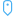# Calculate geometry

Feature geometries can be calculated for line and polygon features. For lines, the length of each feature is calculated. For polygons, the area and perimeter are calculated. The properties can be calculated in meters, kilometers, feet, or miles.

Your dataset must have a location field before you can calculate geometries. You can add a location field to your dataset using Enable Location.

## Calculate geometry for your dataset

Use the following steps to calculate geometry:

1. Expand the dataset for which you will calculate feature size.
2. Click the location field button.
3. Click Select unit and choose a unit from the menu. The units include meters, kilometers, feet, and miles.
4. Click Add length for line features or Add area and perimeter for area features.

The geometry subfields are added below the location field.

## Use geometry fields

Geometry subfields function as number fields, meaning they can be used to style map features, added as numeric variables on charts, or summarized on summary tables. Geometry can also be used to normalize your data by area when you create a choropleth map.

You can also rename a geometry field or use it to apply a dataset filter.

## Export datasets with geometry fields

You can export compatible datasets to CSV, shapefile, or GeoJSON formats. If you export a dataset with geometry fields, a compressed folder is created containing the files in the format you selected. If your folder contains two files, the first file will contain the fields from the original dataset and the second file will contain the geometry fields. The ObjectID field can be used to join the two datasets.